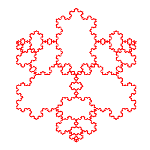# Recursive Fractals: Koch Curve [JAVA]We have seen a first program called “DrawWorld” we introduced the JAVA programming oriented graphics. Programming This module has helped us to see a first recursive fractal: The triangle Sierpisnki.

Let's change this basic program to generate a new basic recursive fractal: The Kuch curve.

It is a fractal that is built recursively from a straight line. Its sides are divided into three equal parts and the central segment is changed by two equal forming 60 degrees between the previous and if.

The Koch curve, also known as snowflake is a fractal that can be obtained by different methods as so-called IFS or Function Systems iterated (Deterministic or), rule-based systems, etc..

The recursive algorithm has also the virtue of representing a concept closely associated with fractals: infinity. The essence of recursion to describe a very simple form of the curve itself. A universe that contains another and this in turn copy the pattern on a smaller scale (so contractive) an endlessly repeating sequence.

Koch curve belongs to the self-similar fractal, be the method of obtaining the deterministic.

## Fractal Dimension

The dimension an object is placed or topological concept that classifies objects metric spaces. The intuitive notion of whole dimensions spaces clashes with the so-called fractal dimensions, taking actual values.

The Peano curve is a curve capable of filling up. Do you have therefore two dimensions?, one wonders.

Is associated with a fractal dimension of roughness, or fragmentation, thereof, so that a greater dimension present a more rough or jagged. In any case gives information about its complexity characterizing.

Koch curve has a ratio s = 1/3, with n = 4, so its fractal dimension is:

D = ln4/ln3 ~ 1.269

If each of these new segments are again divided recursively curve obtained Hoch

.

If we use three lines, instead of one as an initiator, equilateral triangle shaped form appears classic snowflake, name with which this configuration is known fractal.

## Generator algorithm

We have defined a function “paintRecursivo” (I called from the method “paint”) spent the points of the line or lines of the triangle, and the recursion. The function computes the vertices of the new segments, paints the figure and calls itself again reducing the recursion.

Therefore, on each call to the function reduces the value of recursion, so that when it is zero finishes carrying recursion.

```import java.applet.Applet;
import java.awt.Graphics;
/**
* @ Author José Juan Aliaga
*/
public class MainApp extends Applet {
double xp1 = 300;
double p1 = 300;
double xp2 = 10;
double p2 = 300;
double sin60 = Math.sin(3.14/3.);

public MainApp() { }

public static void main(String[] args) { }

public void paint(Graphics g){
}

private void paintRecursivo(Graphics g, int i, double XP12, double yp12, double Xp22, double yp22 ) {
double dx =(Xp22-XP12)/3.;
double dy =(yp22-yp12)/3.;
double xx =  3 * XP12 * sin60 dx/2.-dy;
double yp12  3 * y = dy / 2.   dx * sin60;
if(i<= 0){
g.drawLine((int)XP12,(int)yp12,(int)Xp22,(int)yp22);
}
else{
paintRecursivo(g,i-1, XP12, yp12, XP12   dx,yp12   dy);
paintRecursivo(g,i-1   dx XP12,yp12   dy,xx,yy);
paintRecursivo(g,i-1, xx,yy,Xp22-dx,yp22-d);
paintRecursivo(g,i-1, Xp22-dx,yp22-d,Xp22, yp22);
}
} }```